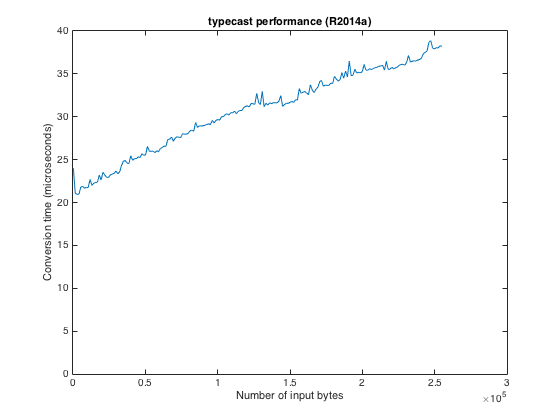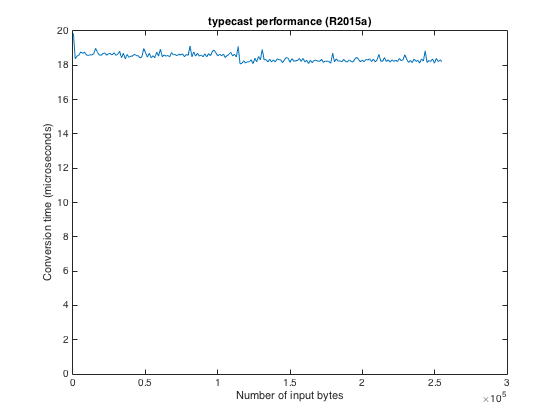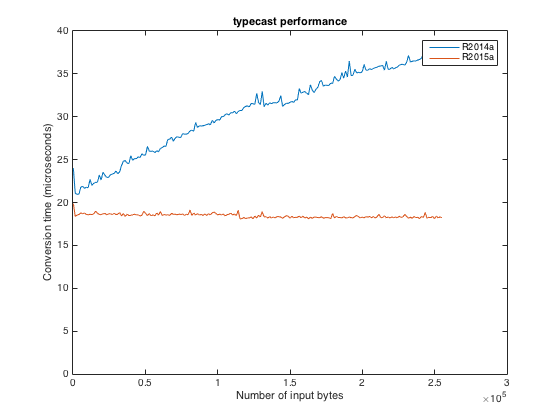# Typecast got faster in R2014b

Some years ago we added the function typecast to MATLAB. I've been wanting to write about this useful little function ever since we significantly speeded it up in the R2014b release.

The function typecast converts numeric values from one type to another based on the underlying bytes in the numeric representation.

Clear as mud, right? Let me try a simple example to illustrate. Here is a vector of two numbers, each of which is represented using one byte:

x = uint8([128 6])

x =

128    6



The call typecast(x,'uint16') takes those two one-byte numbers and converts them to one 16-bit number by jamming together the original bytes.

y = typecast(x,'uint16')

y =

1664



Here's how the number 1664 is related to the original pair of numbers:

128 + 6*2^8

ans =

1664



If you give typecast four one-byte numbers, it will happily convert them into a pair of two-byte numbers.

p = uint8([128 6 200 3])

p =

128    6  200    3


typecast(p,'uint16')

ans =

1664    968



Or, if you prefer, it will convert them into one four-byte number:

typecast(p,'uint32')

ans =

63440512



The function typecast performs these conversions between all of the integer and floating-point numeric types. These days I tend to use typecast (together with its cousin swapbytes) when writing low-level binary file reading code. For files that fit easily into memory, I might just read all the file bytes at once using fileread, and then I'll use indexing, swapbytes, and typecast into meaningful MATLAB data.

Let's examine how long it takes to perform this conversion. First, make a vector containing 256 one-byte values.

bytes = uint8(0:255);


Now use timeit to estimate how long it takes to convert those values to 128 two-byte values.

f = @() typecast(bytes,'uint16');
t_microseconds = timeit(f) * 1e6

t_microseconds =

19.8762



How would you expect the computation time to change as the input vector gets longer?

Here's a plot of computation times that I measured using MATLAB R2014a.

r2014a = load('typecast speedup R2014a ah-eddins-maci');
plot(r2014a.n*256,r2014a.times*1e6)
xlabel('Number of input bytes')
ylabel('Conversion time (microseconds)')
ax = gca;
ax.YLim(1) = 0;
title('typecast performance (R2014a)')The computation time appears to be proportional to the input vector length, which is not too surprising.

And here are the results measured using the latest MATLAB (R2015a).

r2015a = load('typecast speedup R2015a ah-eddins-maci');
plot(r2015a.n*256,r2015a.times*1e6)
xlabel('Number of input bytes')
ylabel('Conversion time (microseconds)')
ax = gca;
ax.YLim(1) = 0;
title('typecast performance (R2015a)')To make the comparison easier, show the results on the same plot.

plot(r2014a.n*256,r2014a.times*1e6)
hold on
plot(r2015a.n*256,r2015a.times*1e6)
xlabel('Number of input bytes')
ylabel('Conversion time (microseconds)')
ax = gca;
ax.YLim(1) = 0;
title('typecast performance')
legend({'R2014a','R2015a'})Now that is a little surprising! Not only is the R2015a computation time lower, it appears to be independent of the input vector length. That's because the implementation has been optimized to avoid making a copy in memory of the input vector's data. (For more about this kind of optimization, see Loren's 2006 blog post about MATLAB memory use for functions and variables.)